# NA Function

Returns the #N/A error value from a calculation

## What is the NA Function?

The NA Function is categorized under Excel Information functions. It will return the #N/A error value. N/A means “no value available” or “not available.”

As a financial analyst, the NA function can be used to mark empty cells and thus avoid the inclusion of empty cells in the calculation. It can also be used to highlight cells where information is missing.

### Formula

=NA()

The NA function does not require an argument.

### How to use the NA Function in Excel?

To understand the uses of the NA function, let’s consider a few examples:

#### Example 1

Suppose we are given the following data: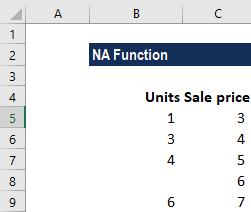In the data above, we are aware that certain cells are blank. To find out the total amount instead of using the formula Units * Sales price, we will use the formula: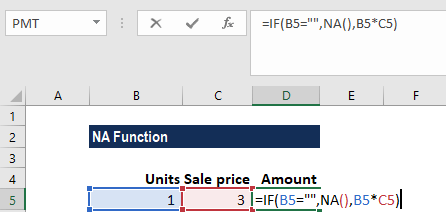We get the result below: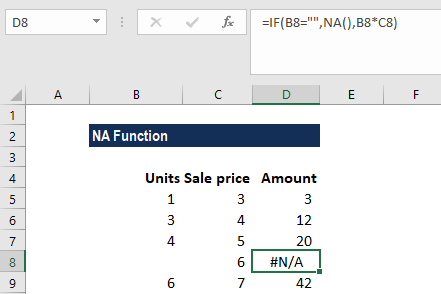Now, if the quantity is missing and there isn’t enough data to run the calculation, the formula checks to see if the cell in column B is blank. If it is, it returns the #N/A error using the NA function. If a value exists, the normal calculation would be run.

#### Example 2

When we are given large datasets, VLOOKUPs can be slow to run. In such a scenario, we can use the NA function and two VLOOKUPs to complete things faster.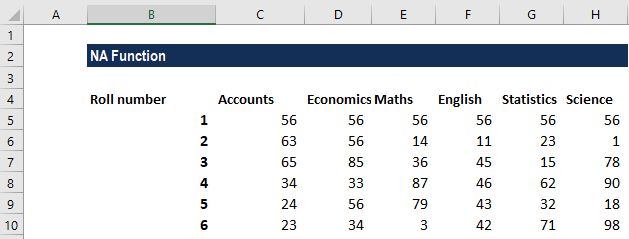Suppose the data above ran into a million rows. In such a scenario, the simple VLOOKUP would be painfully slow. Instead, we can use the formula below: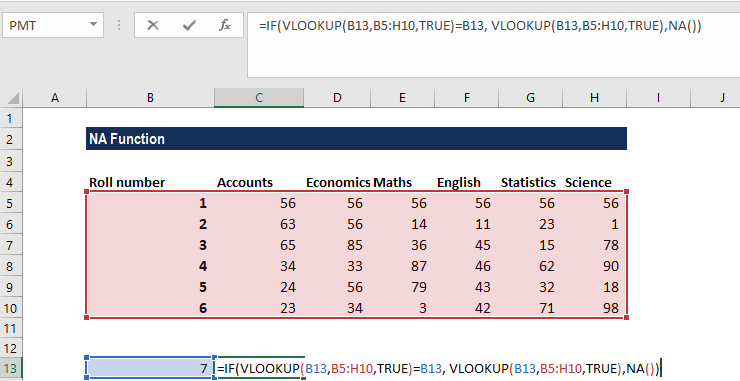We get the result below: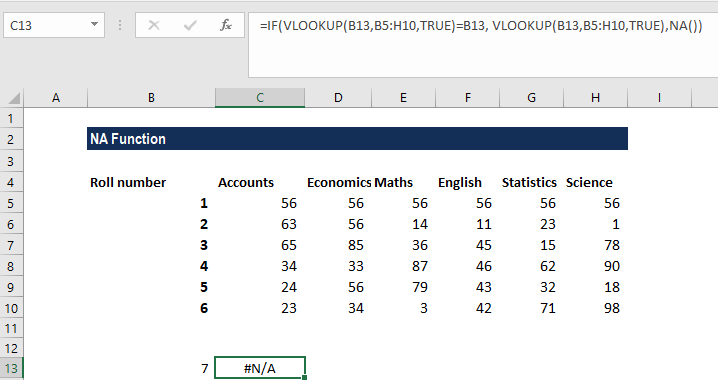In the formula above, we used NA() so we get an #N/A error, but we can also return a message like “Missing” or “Not found,” as shown below: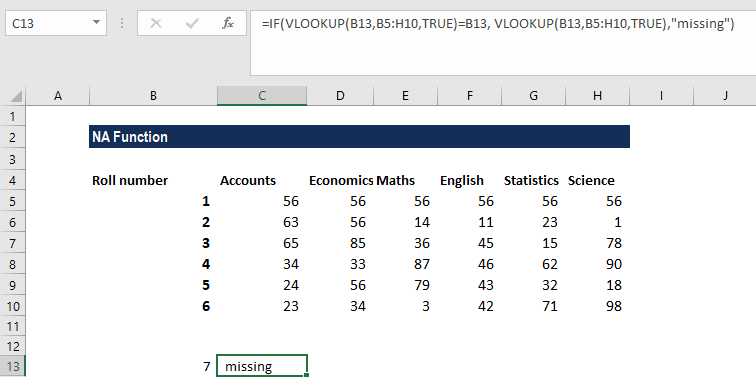For the formula to work, we need to ensure that the data is sorted by the LOOKUP value. So, the roll numbers in the data above are sorted accordingly.

### A few things to remember about the NA Function

1. We need to include empty parentheses along with the function name. If not included, MS Excel will not recognize it as a function.
2. The NA function was provided for compatibility with other spreadsheet programs.
3. When other formulas refer to cells that contain #N/A, they also return #N/A.

Thanks for reading CFI’s guide to important Excel functions! By taking the time to learn and master these Excel functions, you’ll significantly speed up your financial analysis. To learn more, check out these additional CFI resources:

• Excel Functions for Finance